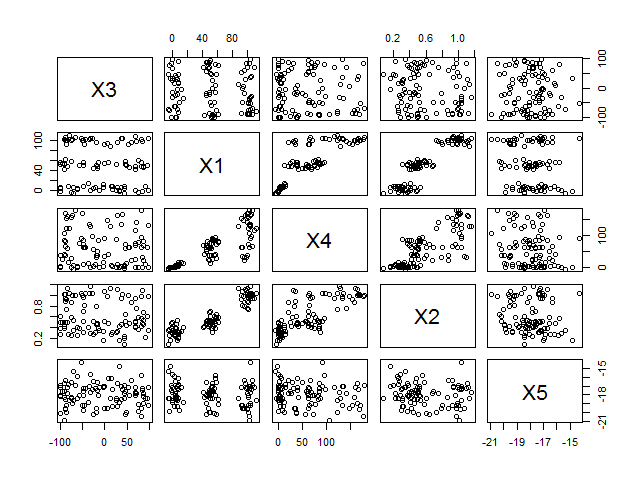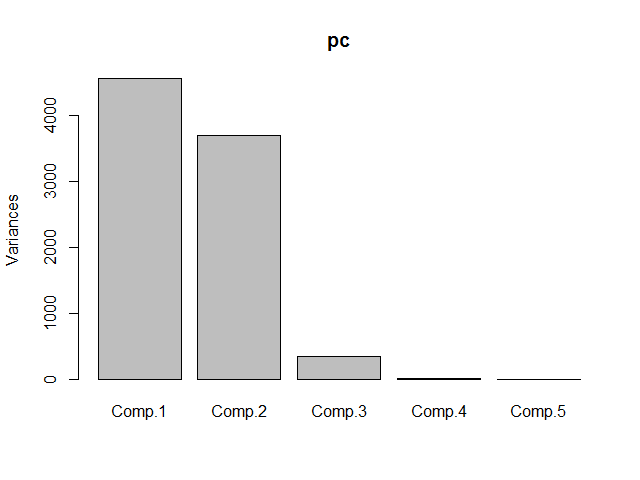# Principal Component Analysis in R

I wanted to know a little more on Principal Component Analysis (PCA) in R. For this purpose, I first created my own artificial dataset. I wanted to reuse the same dataset later on for performing also cluster analysis, so I put a little bit of thought in how to create it.

This is the R code I used.

Let’s go through this step by step.

First, I created a vector of length 100 containing values 1, 2 or 3: `Classes <- sample(1:3, 100, replace = TRUE)`. The value would indicate later whether a certain observation in my dataset belongs to one of three different clusters of data.

Next, I created a function that can be used to create a dataset automatically. The created dataset has five different variables X1 to X5. X3 is a uniformly distributed random variable in the range of -100 to 100: `X3 <- sample(-100:100, n, replace=TRUE)`. X5 is normally distributed random variable with mean -18 and a standard deviation of 1.2: X5 <- rnorm(n, -18, 1.2). In other words, X3 and X5 are totally unrelated to all other variables. In contrast, X1, X2 and X4 are all correlated to each other. An observation is placed in one of three different clusters of observations along three dimensions denoted as X1, X2 and X4. X1 has three cluster centers at 3, 50 and 100 and a standard deviation of 5: `X1 <- rnorm(n, means1[class], sd1)`; X2 has three cluster centers at 0.3, 0.5 and 1.0 and a standard deviation of 0.1: `X2 <- rnorm(n, means2[class], sd2)`; X4 is derived from X1: `X4 <- X1 + X1 * runif(n, -0.8, 0.8)`. Note that I reordered all variables somewhat to make our task a little juicier.

Here’s the correlation matrix and the correlogram.

 X3 X1 X4 X2 X5 X3 1.00000000 -0.1133516 -0.08752043 -0.09320869 -0.02701275 X1 -0.11335158 1.0000000 0.83200505 0.90965123 -0.14800759 X4 -0.08752043 0.8320051 1.00000000 0.75789451 -0.15882644 X2 -0.09320869 0.9096512 0.75789451 1.00000000 -0.13261305 X5 -0.02701275 -0.1480076 -0.15882644 -0.13261305 1.00000000It should already be obvious from both the correlation matrix and the correlogram that there is a strong connection between X1, X2 and X4, whereas X3 and X5 are unrelated to the rest. Let’s see whether we can confirm this with a PCA. We use the `princomp` function with the default parameters.

Importance of components: Comp.1 Comp.2 Comp.3 Comp.4 Comp.5
Standard deviation 67.5243440 60.8182793 18.54506263 1.2692048845 1.216295e-01
Proportion of Variance 0.5299356 0.4299031 0.03997229 0.0001872259 1.719413e-06
Cumulative Proportion 0.5299356 0.9598388 0.99981105 0.9999982806 1.000000e+00

The table shows that already component 1 and 2 together capture 96% of the data’s variance. The screeplot confirms this.To find out which components match to which variables, we’ll use the `loadings` function.

</table>
X3 0.596 0.803
X1 -0.459 0.322 0.828
X4 -0.659 0.501 -0.560
X2 1.000
X5 1.000
</tr>
Comp.1 Comp.2 Comp.3 Comp.4 Comp.5
Proporation Var 0.2 0.2 0.2 0.2 0.2
Cumulative Var 0.2 0.4 0.6 0.8 1.0
Component 4 and 5 map to variables X2 and X5. They are clearly independent from the rest. Component 1, 2 and 3 map to variables X3, X1 and X4. Wait a second. Does this make sense? Something is fishy here. From how we constructed the sample dataset, we would actually expect variables X1, X2 and X4 to belong together - not X1, X3 and X4! Did we maybe make a mistake somewhere? Unfortunately we did. `princomp` by default uses the variance matrix - not the correlation matrix - to compute the different components. Variances unlike correlations, however, depend strongly on the scale and units of the input data. This means, either __[we must scale our input data prior to performing a principal component analysis or we must base our analysis on the correlations, not the variances, between our variables](http://stats.stackexchange.com/questions/53/pca-on-correlation-or-covariance)__. Let's quickly have a look at the variance matrix.
 X3 X1 X4 X2 X5 X3 4043.545354 -287.916544 -307.35406 -1.74489036 -2.22236234 X1 -287.916544 1595.569658 1835.40627 10.69703439 -7.64903703 X4 -307.354064 1835.406265 3049.97811 12.32218479 -11.34843658 X2 -1.744890 10.697034 12.32218 0.08666848 -0.05051051 X5 -2.222362 -7.649037 -11.34844 -0.05051051 1.67390100
Note how very different the result looks compared to the correlation matrix above. For example (in absolute numbers) the variance between X1 and X3 is much higher than the variance between X1 and X2, although we know for sure that the relation should actually be inverse. Looking at the correlation matrix, the relations make much more sense. Fortunately, it's pretty easy to perform a PCA based on the correlation matrix by using the `cor = TRUE` parameter in the `princomp` function.
Importance of components: Comp.1 Comp.2 Comp.3 Comp.4 Comp.5
Standard deviation 1.649629 1.013166 0.9597193 0.50290695 0.27972026
Proportion of Variance 0.544255 0.205301 0.1842122 0.05058308 0.01564869
Cumulative Proportion 0.544255 0.749556 0.9337682 0.98435131 1.00000000
![Screeplot](/public/img/2015-05-31-screeplot2.png "Screeplot") Looking at the summary output and the screeplot, we'd probably conclude that having three components would be a good choice. Let's look at the loadings.